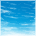# Hi, I have a problem when I tried to convert the data typ...

cancel
Showing results for
Did you mean:

## Hi, I have a problem when I tried to convert the data typ...

1,853 ViewsContributor II
Hi, I have a problem when I tried to convert the data type. the processor is MCF5235CVM150 and the compiler is diab 5.2 from windriver (vxworks5.5.1). I have written a very simple function to test the type conversion. But the results were not as expected.

{
float fv = 2147470000.0;/*2147483646.0;*/
int iv;

printf("sizeof(float) = %d, fv = %f\n",sizeof(fv),fv);

iv = (int)fv;
printf("int iv = %d\n", iv);

return;
}

and the results are:
sizeof(float) = 4, fv = 2147469952.000000
int iv = 2147469952

when I changed the fv value to 2147483646.0, the results are:
sizeof(float) = 4, fv = 2147483648.000000
int iv = -2147483648

I don't understand whether this is a precision problem or something else caused by compiler.  I really appreciate it if somebody can give me a hint.

I paste also the dissembled code here just for convenience.
{
0x209334       +0x004:                 MOVE  .L    D7,-(A7)
float fv = 2147470000.0;/*2147483646.0;*/
0x209336       +0x006:                 MOVE  .L    #0x4effff95,D0
0x20933c       +0x00c:                 MOVE  .L    D0,(0xfffc,A6)
int iv;

printf("sizeof(float) = %d, fv = %f\n",sizeof(fv),fv);
0x209340       +0x010:                 MOVE  .L    (0xfffc,A6),D0
0x209344       +0x014:                 JSR         fltodb
0x20934a       +0x01a:                 MOVE  .L    D1,-(A7)
0x20934c       +0x01c:                 MOVE  .L    D0,-(A7)
0x20934e       +0x01e:                 MOVEQ       #0x4,D0
0x209350       +0x020:                 MOVE  .L    D0,-(A7)
0x209352       +0x022:                 PEA         _str_cmp + 0xca
0x209358       +0x028:                 JSR         _printf
0x20935e       +0x02e:                 LEA         (0x10,A7),A7

iv = (int)fv;
0x209362       +0x032:                 MOVE  .L    (0xfffc,A6),D0
0x209366       +0x036:                 JSR         fltol
0x20936c       +0x03c:                 MOVE  .L    D0,D7
printf("int iv = %d\n", iv);
0x20936e       +0x03e:                 MOVE  .L    D7,-(A7)
0x209370       +0x040:                 PEA         _str_cmp + 0xe7
0x209376       +0x046:                 JSR         _printf

return;
}
0x20937e       +0x04e:                 MOVE  .L    (0xfff4,A6),D7
0x209382       +0x052:                 UNLK        A6
0x209384       +0x054:                 RTS
0x209386       +0x056:                 NOP

Labels (1)
• ### General

6 Replies
619 ViewsContributor II
Hi, Rich.

But what I don't understand is why the printed float value is also not correct.

The float variable fv has been asigned 2147470000.0, which does not exceed the limit.

when I printed it on the console using printf("sizeof(float) = %d, fv = %f\n",sizeof(fv),fv); the result is
fv = 2147469952.000000

619 ViewsSenior Contributor II
Hi,

The issue is the rounding you can't really avoid.  Not only can (most) integer numbers not be represented exactly in a 32 bit floating point value, also most *real* numbers cannot be represented, either.  Consider your number:

2147470000.00

This is converted to 32 bit floating point as:

4EFFFF95

Which is:

+1 .11111111111111110010101 x 2^30

If you look at the floating point numbers in this area (the density of floating point numbers is not uniform), you will see:

4EFFFF94 = 2147469824.00
4EFFFF95 = 2147469952.00
4EFFFF96 = 2147470080.00

So you can see that for the number you requested (2147470000.00) the compiler had to choose the nearest floating point value (based on its rounding algorithm), and it chose 4EFFFF95.  But once you tried to print it back out, it no longer knew what you requested -- only the real number -- so it printed it out exactly as 2147469952.00.

There are floating point calculators on the web which allow you to do this, such as:

-- Rich

619 ViewsSenior Contributor II

That might clear things up, as well.
619 ViewsContributor II
Thanks a lot, Rich. Now it is quite clear.
619 ViewsSenior Contributor II
Hi,

I might not understand exactly what problem you are having, and which behavior you don't like...

A 4 byte float has less than 4 bytes for the mantissa, so it can't represent (most) 4 byte integers exactly, so rounding (up or down) occurs.

And when you convert from float to back to integer, the floating point value may not be representable in the integer's width at all.

(In your second case, you would have needed a 33 bit signed integer to represent the value.  A 32 bit signed integer can represent the values from +2^31-1 to -2^31.  If you had used an unsigned integer there (and a %u) you probably would have been OK, but you still would have had the rounding in the floating point value.)

-- Rich
619 ViewsContributor II
Thank you so much, rich.  Now I understand what the problem is.

According to C specification, the four bytes single float format is